# NCERT Solutions for Class 8 Maths Chapter 15 Introduction to Graphs Ex 15.3

NCERT Solutions for Class 8 Maths Chapter 15 Introduction to Graphs Ex 15.3

### NCERT Solutions for Class 8 Maths Chapter 15 Introduction to Graphs Exercise 15.3

Ex 15.3 Class 8 Maths Question 1.
Draw the graphs for the following tables of values, with suitable scales on the axes.
(a) Cost of apples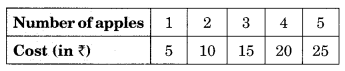(b) Distance travelled by car.(i) How much distance did the car cover during the period 7:30 am to 8 am?
(ii) What was the time when the car had covered a distance of 100 km since its start?
(c) Interest on deposits for a year.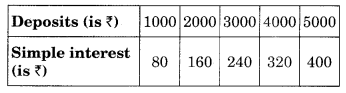(i) Does the graph pass through the origin?
(ii) Use the graph to find the interest on ? 2500 for a year.
(iii) To get an interest of t 280 per year, how much money should be deposited?
Solution: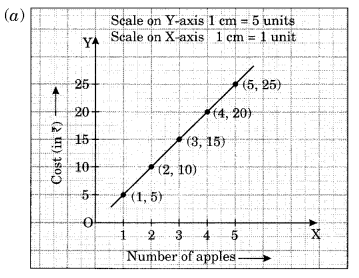(b) (i) The distance covered by the car during the period 7:30 am to 8 am is (120 km – 100 km) = 20 km.
(ii) At 7:30 am, the car had covered a distance of 100 km.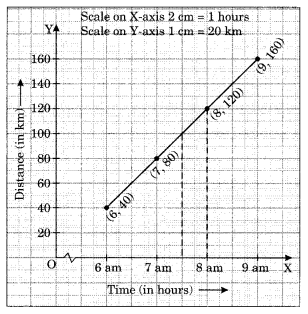(c) (i) Yes, the graph passes through the origin,
(ii The interest on ₹ 2500 is ₹ 200 for 1 year.
(iii) ₹ 3500 should be invested to earn the interest of ₹ 280.Ex 15.3 Class 8 Maths Question 2.
Draw the graph for the following: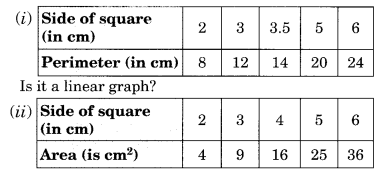Solution: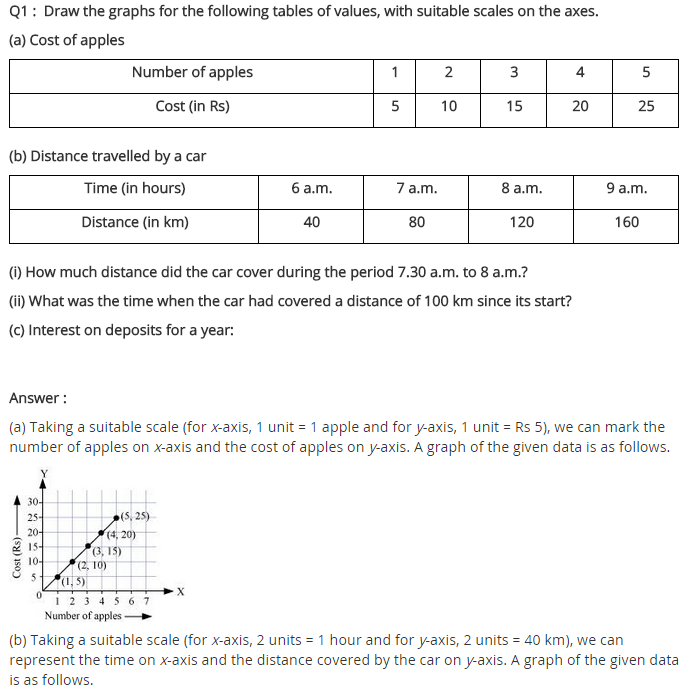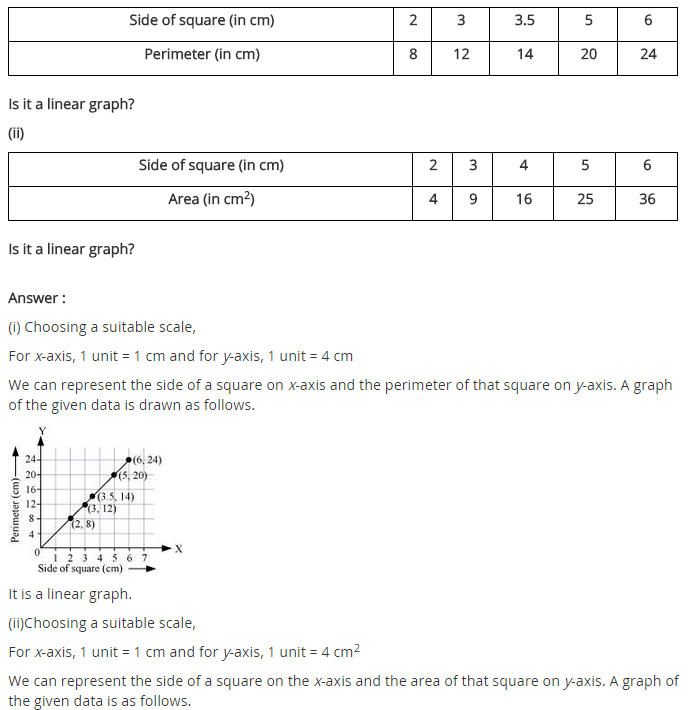## SabDekho

The Complete Educational Website